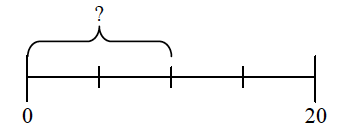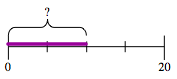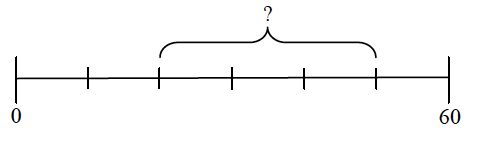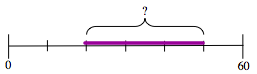### Home > ACC6 > Chapter 2 Unit 10 > Lesson CC2: 2.2.3 > Problem2-65

2-65.

Find the missing lengths in the problems below. Assume each line segment is subdivided into equal segments. Show your work.

1.How much of the entire $20$ unit segment is purple?
Consider the purple part as a portion of the total segment.

1.What is the value of each segment of the $60$ unit line?
After you figure this out, try to find the value of the purple highlighted portion.

$30$ units. Be sure to explain why this is the answer.